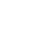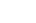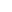# NOTES## TS 10th class maths concept

Studying mathematics successfully meaning that, children take responsibility for their own learning and learn to apply the concepts to solve problems.

This note is designed by the ‘Basic In Maths’ team. These notes to do help students fall in love with mathematics and overcome fear.

## 1. REAL NUMBERS

• Rational number: The number, which is written in the form of p/q where p,q are integers q not equal to zero is called a rational number. It is denoted by Q.

• Irrational numbers:- the number, which is not rational is called an irrational number. It is denoted by Q’ or S.

• Euclid division lemma:- For any positive integers a and b, then q, r are integers exists uniquely satisfying the rules a = bq + r, 0 ≤ r < b.

• Prime number:- The number which has only two factors 1 and itself is called a prime number. (2, 3, 5, 7 …. Etc.)

• Composite number:- the number which has more than two factors is called a composite number. (4, 6, 8, 9, 10,… etc.)

• Co-prime numbers:- Two numbers  said to be co-prime numbers if they have no common factor except 1. [Ex: (1, 2), (3, 4), (4, 7)…etc.]

• To find HCF, LCM by using prime factorization method:  H. C.F= product of the smallest power of each common prime factors of given numbers. L.C.M = product of the greatest power of each prime factor of given numbers.

• In p/q, if prime factorisation of q is in form 2m 5n, then p/q is terminating decimal. Otherwise non-terminating repeating decimal.
• Decimal numbers with the finite no. of digits is called terminating Decimal numbers with the infinite no. of digits is called non-terminating decimal. In a decimal, a digit or a sequence of digits in the decimal part keeps repeating itself infinitely. Such decimals are called non-terminating repeating decimals.

• ‘p’ is a prime number and ‘a’ is a positive integer, if p divides a2, then p divides a.

• If ax = N then x =

(i) log (xy) = log(x) + log(y)  (ii) log (x/y) = log( x) – log( y) (iii) log (xm ) = m log (x)Loading...Taking too long?Reload document
|Open in new tab

Pair Of Linear Equations In two Variables Concept and Solutions: Click Here

### TS 10th class maths concept

Visit My Youtube Channel: Click on Below Logo## ICSE IX Class Maths Concept

ICSE IX Class Maths Concept: This note is designed by the ‘Basics in Maths’ team. These notes to do help the ICSE 9th class Maths students fall in love with mathematics and overcome their fear.

These notes cover all the topics covered in the ICSE 9th class Maths syllabus and include plenty of formulae and concept to help you solve all the types of ICSE 9th

Math problems are asked in the CBSE board and entrance examinations.

## 1. RATIONAL AND IRRATIONAL NUMBERS

Natural numbers: counting numbers 1, 2, 3… called Natural numbers. The set of natural numbers is denoted by N.

N = {1, 2, 3…}

Whole numbers: Natural numbers including 0 are called whole numbers. The set of whole numbers denoted by W.

W = {0, 1, 2, 3…}

Integers: All positive numbers and negative numbers including 0 are called integers. The set of integers is denoted by I or Z.

Z = {…-3, -2, -1, 0, 1, 2, 3…}

Rational number: The number, which is written in the form of, where p, q are integers and q ≠ o is called a rational number. It is denoted by Q.

∗ In a rational number, the numerator and the denominator both can be positive or negative, but our convenience can take a positive denominator.

Ex: – can be written as   but our convenience we can take

Equal rational numbers:

For any 4 integers a, b, c, and d (b, d ≠ 0), we have  ⇒ ad = bc

The order of Rational numbers:

If  are two rational numbers such that b> 0 and d > 0 then  ⇒ ad > bc

The absolute value of rational numbers:

The absolute value of a rational number is always positive. The absolute value of   is denoted by .

Ex: – absolute value of

To find rational number between given numbers:

• Mean method: – A rational number between two numbers a and b is

Ex: – insert two rational number between 1 and 2

1 <   < 2   ⟹     1 <    < 2

1 <  < 2   ⟹   1 <   2

To rational numbers in a single step: –

Ex:- insert two rational numbers between 1 and 2

To find two rational numbers, we 1 and 2 as rational numbers with the same denominator 3

(∵ 1 + 2 = 3)

1 =     and 2 =

Note: – there are infinitely many rational numbers between two numbers.

The decimal form of rational numbers∗ Every rational number can be expressed as a terminating decimal or a non-terminating repeating decimal.

Converting decimal form into   the form:

1. Terminating decimals: –

1.2 =

1.35 =

2. Non-Terminating repeating decimals: –Irrational numbers:

• The numbers which are not written in the form of  , where p, q are integers, and q ≠ 0 are called rational numbers. Rational numbers are denoted by QI or S.
• Every irrational number can be expressed as a non-terminating decimal or non-repeating decimal.

Ex:- and so on.

• Calculation of square roots:
• There is a reference of irrationals in the calculation of square roots in Sulba Sutra.
• Procedure to finding value:##### Visit my Youtube Channel: Click on Below Logo